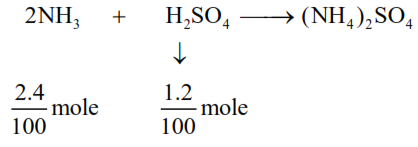# 0.8 g of an organic compound wasQuestion:

$0.8 \mathrm{~g}$ of an organic compound was analysed by Kjeldahl's method for the estimation of nitrogen. If the percentage of nitrogen in the compound was found to be $42 \%$, then $\mathrm{mL}$ of $1 \mathrm{M}$ $\mathrm{H}_{2} \mathrm{SO}_{4}$ would have been neutralized by the ammonia evolved during the analysis.

Solution:

Organic compound : $0.8 \mathrm{gm}$

wt. of $\mathrm{N}=\left(\frac{42}{100} \times 0.8\right) \mathrm{gm}$

mole of $\mathrm{N}=\frac{42 \times 0.8}{100 \times 14}=\frac{2.4}{100} \mathrm{~mol}$

moles of $\mathrm{NH}_{3}=\frac{2.4}{100}$$\frac{1.2}{100}=1 \times \mathrm{V}(\ell)$

$\Rightarrow \mathrm{V}_{\mathrm{H}_{2} \mathrm{SO}_{4}}=\frac{1.2}{100} \ell=12 \mathrm{~m} \ell$